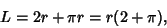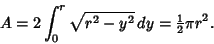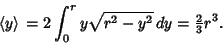## SemicircleHalf a Circle. The Perimeter of the semicircle of Radiusis(1)

and the Area is(2)

The weighted mean ofis(3)

The Centroid is then given by(4)

The semicircle is the Cross-Section of a Hemisphere for any Plane through the z-Axis.

See also Arbelos, Arc, Circle, Disk, Hemisphere, Lens, Right Angle, Salinon, Thales' Theorem, Yin-Yang